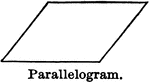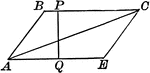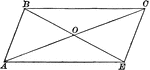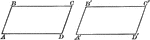### Parallelogram

Illustration of a parallelogram, a quadrilateral which has opposite sides parallel.### Altitude and Diagonal of a Parallelogram

Illustration of a parallelogram with altitude and diagonal drawn.### Parallelogram With Diagonals Bisecting Each Other

Illustration of a parallelogram with diagonals drawn to show they bisect each other.### Equal Parallelograms

Illustration of a two equal parallelograms. Two parallelograms are equal, if two sides and the included…